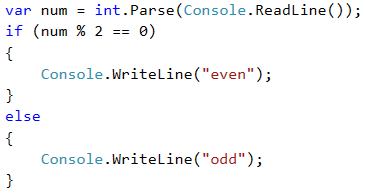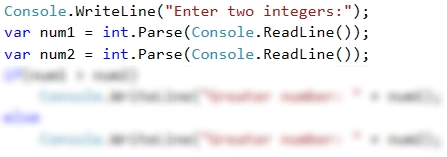If-Else Conditions – Examples

Now let's take a few examples (exercises) to learn how to use if-else conditional statements in practice.

Video: Examples of If-Else

Watch the video lesson about the "Even or Odd Number" and "The Larger Number" problems and their solutions: https://youtu.be/qIUoKiObr-A.

Example: Even or Odd Number

Write a program that checks whether an integer is even or odd.

Hint and Guidelines

We can solve the problem with one if-else statement and the operator %, which returns a remainder by dividing two numbers.Testing in the Judge System

https://judge.softuni.org/Contests/Practice/Index/506#2.

Example: The Larger Number

Write a program that reads from the console two integers and prints the larger of them. Print "Greater number: " + the bigger number.

The input comes as two numbers, each on a separate line. Sample input:

3
5


The output is a message like the shown below. Sample output:

Greater number: 5


Hint and Guidelines

Our first task is to read the two numbers from the console. Then, with a simple if-else statement, combined with the operator for greater than (>), we do check. Part of the code is deliberately blurred, so you can test what you learned so far.